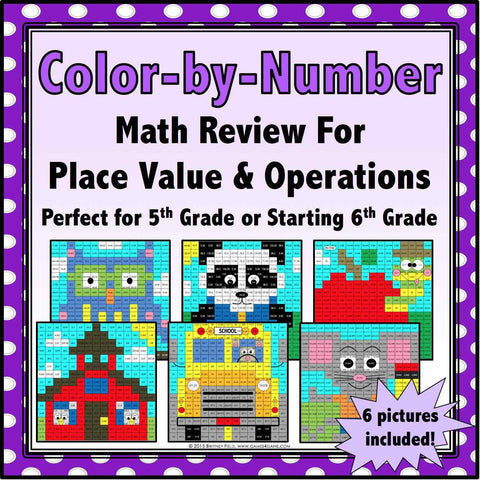## Back to School Color-by-Number - 5th/6th Grade

• \$480

This back to school set comes with 6 "School Days" math color-by-number activities for reviewing and practicing place value and operations standards from 5th grade. This set is perfect to use as review with incoming 6th graders or as practice with 5th graders who have already learned these skills.

Included with this "School Days" color-by-number set:
Instructions
6 color by number worksheets
6 student problem worksheets

Here are the Common Core standards covered by the color-by-number activities:

CCSS.MATH.CONTENT.5.NBT.A.2
Explain patterns in the number of zeros of the product when multiplying a number by powers of 10, and explain patterns in the placement of the decimal point when a decimal is multiplied or divided by a power of 10.

CCSS.MATH.CONTENT.5.NBT.A.3.A
Read and write decimals to thousandths using base-ten numerals, number names, and expanded form.

CCSS.MATH.CONTENT.5.NBT.A.4
Use place value understanding to round decimals to any place.

CCSS.MATH.CONTENT.5.NBT.B.5
Fluently multiply multi-digit whole numbers using the standard algorithm.

CCSS.MATH.CONTENT.5.NBT.B.6
Find whole-number quotients of whole numbers with up to four-digit dividends and two-digit divisors.

CCSS.MATH.CONTENT.5.NBT.B.7
Add, subtract, multiply, and divide decimals to hundredths.